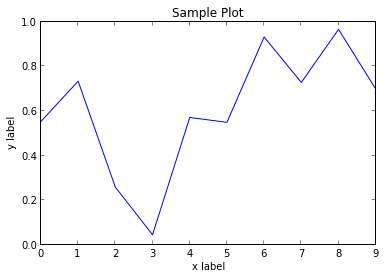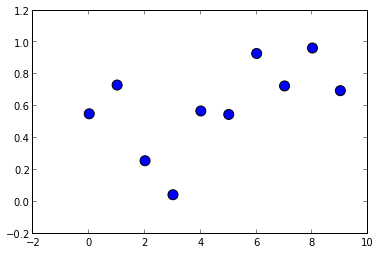# Python¶

In :
print("Hello World")

Hello World

In :
x = 1
y = x + 2
print x, y

1 3


# Libraries¶

## NumPy¶

The NumPy library provides multi-dimensional arrays (e.g. vectors, matrices, ...)

NumPy is usually referred to using the shorthand module name np:

In :
import numpy as np

In :
v = np.zeros(3)
M = np.ones((3,4))
A = np.array([[1,2,3],[4,5,6]])
print "v\n", v
print "M\n", M
print "A\n", A

v
[ 0.  0.  0.]
M
[[ 1.  1.  1.  1.]
[ 1.  1.  1.  1.]
[ 1.  1.  1.  1.]]
A
[[1 2 3]
[4 5 6]]


You can also work with sub-matrices in a similar fashion as in MatLab, but starting with index 0:

In :
print A
print "the first column of A:", A[:,0]
print "the first    row of A:", A[0,:]

[[1 2 3]
[4 5 6]]
the first column of A: [1 4]
the first    row of A: [1 2 3]

In :
x = np.arange(10)
print x

[0 1 2 3 4 5 6 7 8 9]

In :
y = np.random.uniform(size=10) # note the named argument here. The 1st (default) arguments are 0 and 1.
y # last element in cell is printed automatically

Out:
array([ 0.552715  ,  0.73310284,  0.25826838,  0.04512618,  0.57048334,
0.54865524,  0.93093535,  0.72744663,  0.96537373,  0.69774996])

## Matplotlib¶

This is a library used to plot graphs conveniently directly from Python. Many graph types are supported.

In :
import matplotlib.pyplot as plt

In :
from IPython.display import HTML
HTML('<iframe src=http://matplotlib.org/gallery.html width=700 height=350></iframe>')

Out:
In :
plt.plot(x, y)
plt.title("Sample Plot")
plt.xlabel("x label")
plt.ylabel("y label");In :
plt.scatter(x, y, s=100) # press TAB after opening parenthesis to see help on scatter;## Scikit-Learn¶

In :
from IPython.display import HTML
HTML('<iframe src=http://scikit-learn.sourceforge.net/dev/auto_examples/index.html width=900 height=350></iframe>')

Out:

Easy-to use machine-learning with many examples and documentation.

## Classification¶

• Logistic Regression
• Support Vector Machines
• Perceptron
• Decision Trees
• Random Forests
• Naive Bayes
• Neares Neighbors
• ...

## Regression¶

• Linear Regression
• Lasso
• Lars
• Ridge Regression
• Support Vector Regression
• Regression Forests
• ...

## Feature Extraction¶

• Principal Component Analysis
• Independend Component Analysis
• Nonnegative Matrix Factorization
• Sparse Decomposition
• Locally Linear Embedding
• KernelPCA
• ...

## Clustering¶

• $k$-Means
• Mean Shift
• Spectral Clustering
• Affinity Propagation
• DBSCAN
• ...
In [ ]: